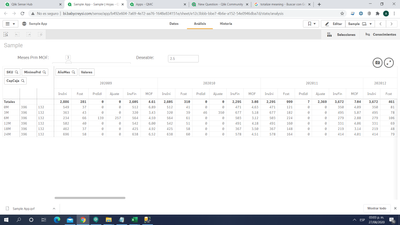# App Development

Announcements
April 22, 2PM EST: Learn about GeoOperations in Qlik Sense SaaS READ MORE
cancel
Showing results for
Did you mean:Contributor III

## Totalize rollforward measure in pivot table

Hi all,

I've been trying to totalize two specific measures in a pivot table, one of them involving a rangemax and the other involving an if. As expected, the calculated total is the formula of the measure applied to the total of the other measures.

I am aware that the way to achieve this is to use Aggr function over the dimensions, but this don't seem to work as my not totalized measures rely on results from the other measures, which depend on the results of the previous period (end of period final measure result is beggining of period first measure result).

Formulas are:

InvIni = If(AñoMes = \$(sAñoMes), \$(vInv), Before([InvFin]))

PrdIdl = RangeMax(\$(cDeseable) * \$(vFcstPrm) - [InvIni] + [Fcst], 0)

Ajuste = If([PrdIdl], Ceil(RangeMax([PrdIdl], Sum(MinimoPrd)) / Sum(CapCaja)) * Sum(CapCaja) - [PrdIdl], 0)

InvFin = [InvIni] + [PrdIdl] + [Ajuste] - [Fcst]

sAñoMes and cDeseable are constants

vFcstPrm is a weighted average of Sum(Cantidad) by AñoMesAttached you can find a sample of the problem I have.

Labels (3)

• ### Qlik Sense

0 RepliesTags
Community Browser